Intervals

Chapter 1 Class 11 Sets
Concept wise

We can write

2 < x < 5

as x ∈ (2, 5)

This is called interval notation

There are different types of intervals

1. Open Interval (a < x < b)
2. Closed interval (a ≤ x ≤ b )
3. Semi Open Interval (a ≤ x < b and a < x ≤ b)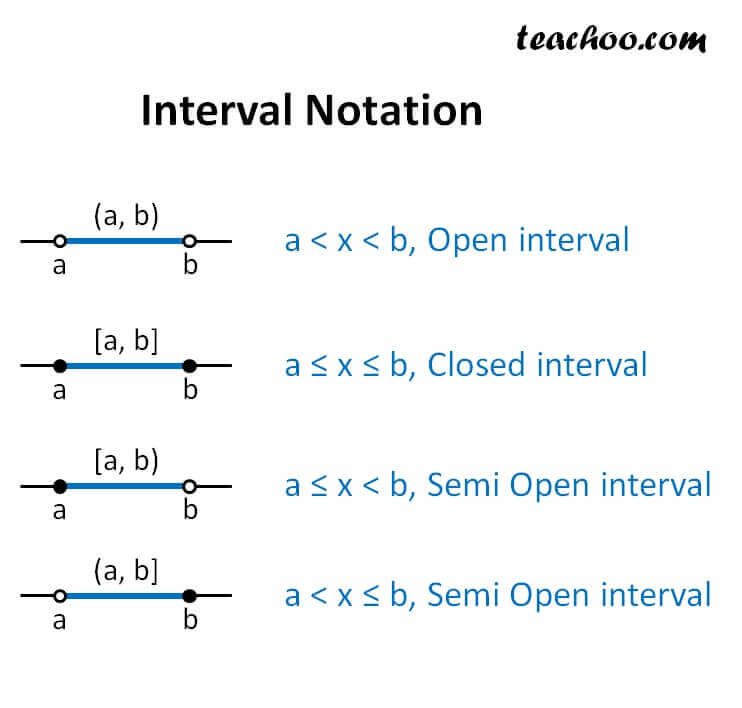## Write x > 2 in interval notation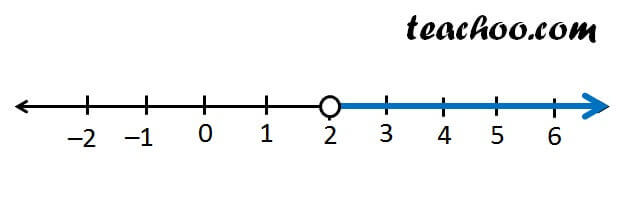So, x goes 2 to infinity

x ∈ (2, ∞)

Note- ∞ and -∞ always has an open bracket

## Write x ≥ 3 in interval notation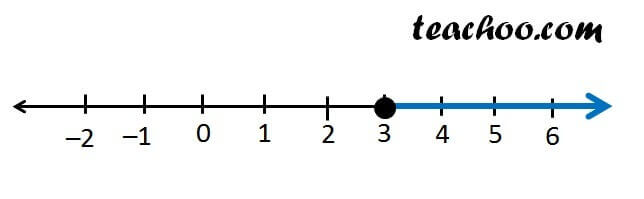So, x goes 3 to infinity

x ∈ [3, ∞)

## Write x < 5 in interval notation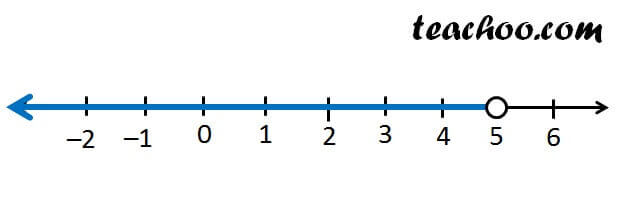So, x goes –infinity to 5

x ∈ (–∞, 5)

Note- ∞ and -∞ always has an open bracket

## Write x ≤ –1 in interval notation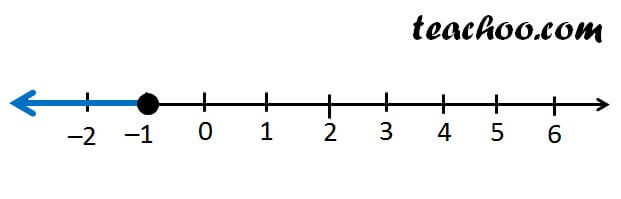So, x goes –infinity to –1

x ∈ (–∞, –1]

## Write x ≤ -1 & x > 2 in interval notation

In this, we have two notations

x ≤ –1

and x > 2

We merge both graphs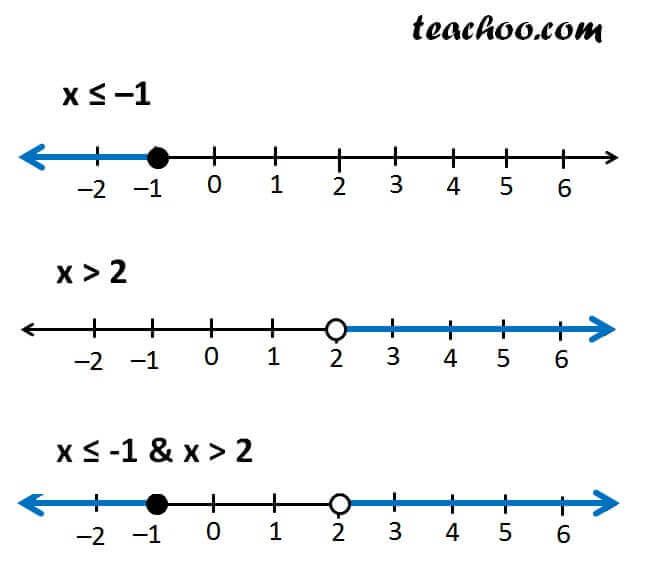So, x goes –infinity to –1 and from 2 to infinity

So, in interval notation, we write it as

x ∈ (–∞, –1] ∪ (2, ∞)

## Write x < 5 & x > 2 in interval notation

In this, we have two notations

x < 5

x > 2

We merge both graphs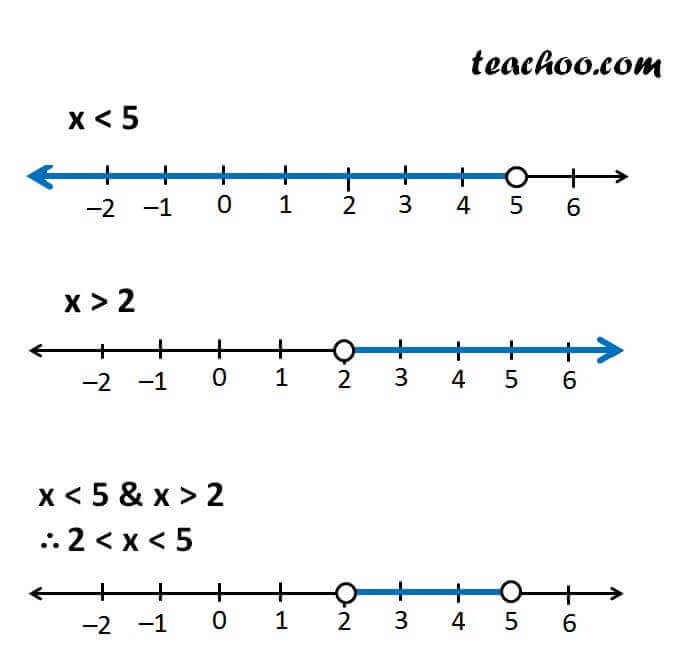So, x goes 2 to 5

So, in interval notation, we write it as

x ∈ (2, 5)

Get live Maths 1-on-1 Classs - Class 6 to 12